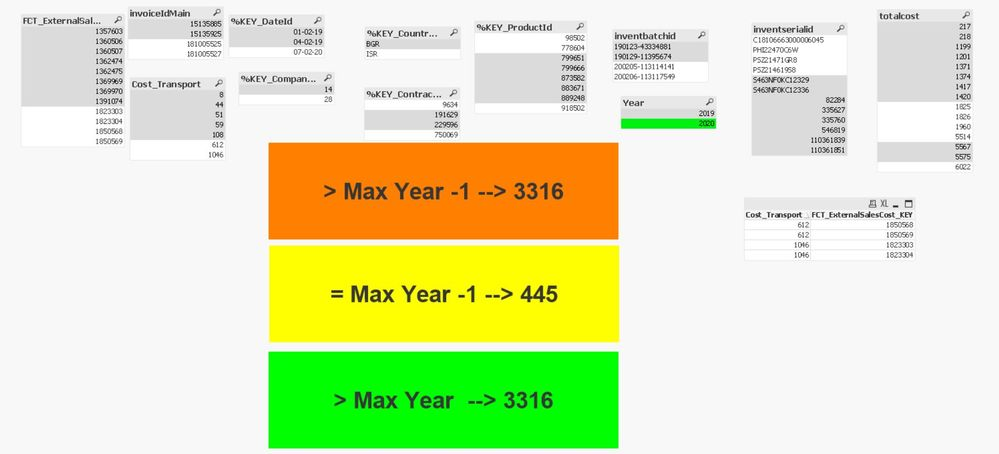# QlikView App Dev

Discussion Board for collaboration related to QlikView App Development.

Announcements
Join us at the Cloud Data and Analytics Tour! REGISTER TODAY
cancel
Showing results for
Search instead for
Did you mean:Creator

## Sum and aggr for PY

Hi to all,

Could you help me with this?

sum(aggr(Cost_Transport, FCT_ExternalSalesCost_KEY))

it returns the cost as it should but i need previous year cost

so  I tried use this:

sum( {<Year{'2018'}>} aggr(Cost_Transport, FCT_ExternalSalesCost_KEY))

but it doesn't work the value is 0

I have  tried some solutions from forum but nothing works.

12 RepliesMaster II

you already have the FCT_ExternalSalesCost_KEY field that's uniqu, so

if I select 2020 (Max year) : total of Cost_Transport from 2019 grouped by FCT_ExternalSalesCost_KEY when we select Year 2020

``sum({<Year={"> \$(=Max(Year)-1)"}>}  aggr(Max({<Year={"> \$(=Max(Year)-1)"}>} Cost_Transport),FCT_ExternalSalesCost_KEY))``

Current selected Year -> Maxyear

``sum({<Year={"\$(=Max(Year))"}>}  aggr(Max({<Year={"\$(=Max(Year))"}>} Cost_Transport),FCT_ExternalSalesCost_KEY))``

Year-1 : If I selected 2020 return 2019 value :

``sum({<Year={"\$(=Max(Year)-1)"}>}  aggr(Max({<Year={"\$(=Max(Year)-1)"}>} Cost_Transport),FCT_ExternalSalesCost_KEY))``

and so on.

output :attached qvw file

Regards,
Taoufiq ZARRA

"Please LIKE posts and "Accept as Solution" if the provided solution is helpful "

(you can mark up to 3 "solutions") 😉Creator
Author

Thank you for sating with me and helping find the right solution.

It works as I need it.

Well.. so many years in QV and still learning 😉Master II

we're all learners 😉

Regards,
Taoufiq ZARRA

"Please LIKE posts and "Accept as Solution" if the provided solution is helpful "

(you can mark up to 3 "solutions") 😉